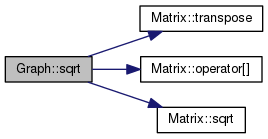NeuroLab
Graph Class Reference

The graph of a function. More...

Inheritance diagram for Graph:[legend]

## Public Member Functions

virtual double getSum ()
Sum of elements. More...

Graphmultiply (double d)
Multiply with a scalar. More...

Graphexp ()
Exponential. More...

Graphsqrt ()
Sqare root for each element. More...

Graphintegrate ()
Integrate. More...

Graphdifferentiate ()
Differentiate. More...

Graphinverse ()
Calculate the inverse. More...

void sortX (int start=0, int end=-1)
Sort the points according to the x values. More...Public Member Functions inherited from Matrix
Matrix ()
default constructor: constructs a zero-dimensional matrix (a scalar).

Matrix (int i)
constructs a one-dimensional matrix

Matrix (int i, int j)
constructs a two-dimensional matrix

Matrix (int i, int j, int k)
constructs a three-dimensional matrix

Matrix (int i, int j, int k, int l)
constructs a four-dimensional matrix

Matrix (int dimensions, int *sizes)
constructs an n-dimensional matrix

virtual ~Matrix ()
destructs the matrix

MatrixsetName (const string &)
set name

string sName ()
get name

int nDimension ()
Get number of dimensions.

int nSize (int n)
Get size for dimension.

Unit getUnit (int n)
Unit of dimension n.

void setPhysical (int n, Physical p)
Set physical dimension of dimension n. More...

void setPhysical (Physical p)
Set main physical dimension. More...

Physical pPhysical (int n)
Get physical dimension for dimension n. More...

Matrix operator[] (int i)
retrieve slices or values More...

Matrixremove (vector< int >)
remove one or more dimensions More...

void operator++ (int i)
increment a zero-dimensional matrix

void operator= (double d)
assignment More...

Matrixoperator+= (const Matrix &)

Matrix operator() (int i, int j)
retrieve ranges - not implemented yet! More...

double to_d ()
convert to double More...

double getMaxValue ()
get maximal value

double getMinValue ()
get minimal value

void replaceNan (double d)
Replaces NaNs. More...

Matrixtranspose ()
Transpose matrix. More...

Matrixoperator= (const Matrix &m)
assignment

Matrix (const Matrix &m)
Copy constructor. More...Public Member Functions inherited from Physical
Physical ()
Construct.

Physical (const Physical &)
Copy.

Physical (string name)
Construct.

Physical (string name, string unitPrefix, string unitSymbol)
Construct.

Physical (string name, Unit unit)
Construct.

virtual string getDescription ()
Retrive the physical name. More...

virtual string getPhysicalDescription ()
Returns the physical description. More...

virtual string getUnitName ()
Returns the unit name.

virtual string getUnitSymbol ()
Returns the unit name.

virtual void setDescription (string name)
Set the name. More...

virtual void setPhysicalDescription (string name)
Set the name. Same as setDescription(). More...

virtual void setUnit (Unit u)
Set the unit.

virtual void setUnitPrefix (int n)
Set unit prefix. More...

virtual Unit getUnit () const
Retrieve the unit.

## Detailed Description

The graph of a function.

A graph is the set of points p, with p = f(a), a element A, where A is the domain of f. This class is a matrix (containing a numerical representation of the graph) with dimension n+1, where n is the number of inputs to f. It is organized so that file << graph appends gnuplot-plottable matrix notation to 'file'. If used as a matrix, you have (for a function with one parameter) graph[i] is the i'th data point, and graph[i] is the input a_i, and graph[i] is the output f(a_i). The main difference to the normal matrix class is that all mathematical operations (integrate, differentiate, multiply, etc.) only work on the output values (i.e. on graph[..]).

Todo:
Only two-dimensional graphs are implemented yet. Change this.

## Member Function Documentation

 Graph& Graph::add ( double d )
inlinevirtual

Adds a scalar to each element of the graph. A reference to the graph itself is returned for convenience, so that you can write things like m.add( a ).multiply( b )

Reimplemented from Matrix.

Here is the call graph for this function: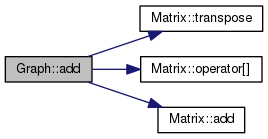Graph& Graph::differentiate ( )
inlinevirtual

Differentiate.

This function differentiates over the last dimension of the graph. A difference-graph is produced, where m' = 0.0, m' = m-m, m'[n] = m[n]-m[n-1]. Doing this on an integrated graph, m.integrate().differentiate(), restores the original graph, but m will be lost (set to 0.0).

Reimplemented from Matrix.

Here is the call graph for this function:Graph& Graph::exp ( )
inlinevirtual

Exponential.

Transforms each element into its exponential. A reference to the graph itself is returned for convenience, so that you can write things like m.exp().multipliy( a ).add( b )

Reimplemented from Matrix.

Here is the call graph for this function: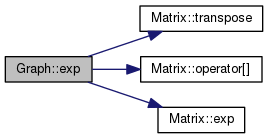virtual double Graph::getSum ( )
inlinevirtual

Sum of elements.

This returns a sum of all elements.

Reimplemented from Matrix.

Here is the call graph for this function: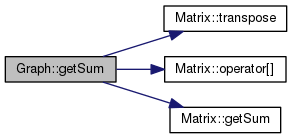Graph& Graph::integrate ( )
inlinevirtual

Integrate.

This function integrates over the last dimension of the graph. A cumulative sum is produced, where m' = m, m' = m+m, m'[n] = m + m + ... + m[n]. Doing this on a differentiated graph, m.differentiate().integrate(), retrieves the original graph, shifted so that m = 0.0, and adding the original m restores the original graph.

Reimplemented from Matrix.

Here is the call graph for this function: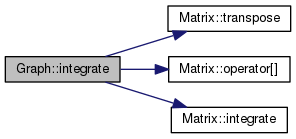Graph& Graph::inverse ( )
inline

Calculate the inverse.

This function just swaps x and y of the graph, and calls sortX() so that all x values are in ascending order afterwards.

Here is the call graph for this function: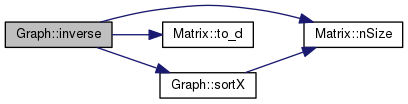Graph& Graph::multiply ( double d )
inlinevirtual

Multiply with a scalar.

Multiplies the graph with a scalar. A reference to the graph itself is returned for convenience, so that you can write things like m.multipliy(3).add(4)

Reimplemented from Matrix.

Here is the call graph for this function:void Graph::sortX ( int start = `0`, int end = `-1` )
inline

Sort the points according to the x values.

This function is used by the inverse() function, after swapping x and y. It sorts all points of the graph, so that the x values of all points will be in ascending order. The currently implemented method is merge sort.

Here is the call graph for this function:Graph& Graph::sqrt ( )
inlinevirtual

Sqare root for each element.

Transforms each element into its square root. A reference to the graph itself is returned for convenience, so that you can write things like m.sqrt().multipliy( a ).add( b )

Reimplemented from Matrix.

Here is the call graph for this function: